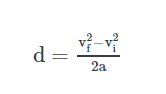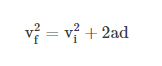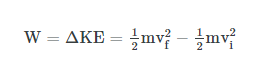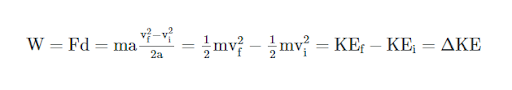# Derivation Of Work Energy Theorem

The derivation of the work-energy theorem is provided here. The work-energy theorem also known as the principle of work and kinetic energy states that the total work done by the sum of all the forces acting on a particle is equal to the change in the kinetic energy of that particle. This explanation can be extended to rigid bodies by describing the work of rotational kinetic energy and torque.

## What is Kinetic Energy?

Kinetic energy is the energy possessed by an object due to its motion or movement. In simple words, it is the energy of motion, observable as the movement of an object, particle, or set of particles.

## Work-Energy Theorem Derivation

The work ‘W’ done by the net force on a particle is equal to the change in the particle’s kinetic energy (KE).Check the detailed work-energy theorem derivation given below.

Let us consider a case where the resultant force ‘F’ is constant in both direction and magnitude and is parallel to the velocity of the particle. The particle is moving with constant acceleration along a straight line. The relationship between the acceleration and the net force is given by the equation “F = ma” (Newton’s second law of motion), and the particle’s displacement ‘d’, can be determined from the equation:Obtaining,The work of the net force is calculated as the product of its magnitude (F=ma) and the particle’s displacement. Substituting the above equations yields:Stay tuned with BYJU’S to get the detailed step-by-step derivation of various topics and understanding the concept easily.

Test your knowledge on Work energy theorem derivation

#### 1 Comment

1. Prasasti Mandal

Thank you Byjus for cleaning my doubt.